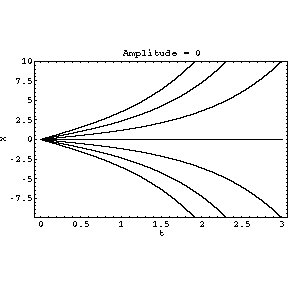# Stochastic stabilisation

Contrary to intuition, high frequency noise tends to stabilise motion. Here we solve the differential equation

x''(t)=(1+Af(t))x(t)

with initial conditions x(0)=0, x'(0)=v with v=-3,-2,-1,0,1,2,3 giving the separate curves. f(t) is the noise, given by the sum of 100 terms of the form cos(t+) with angular frequencygiven randomly in the interval (100,500) and phasegiven randomly in the interval (0,2). The amplitude A increases from 0 to 1000 throughout the animation.

When A=0, the solution is simply x=v sinh t, which grows exponentially. However as A is increased, the solution stabilises to roughly sinusoidal oscillations.

This equation has many applications, for example it describes small oscillations of the stochastic Kapitsa pendulum, in which an inverted pendulum is stabilised by randomly shaking the pivot. The 1 in the equation denotes the unstable effect of gravity in this instance. At present, I am mostly considering cosmological applications, in which photon trajectories in the cosmic microwave background and from distant galaxies may be stabilised and focussed by gravitational lensing due to the intervening lumpy matter distribution. See the paper Stochastic stabilization of cosmological photons for more details.